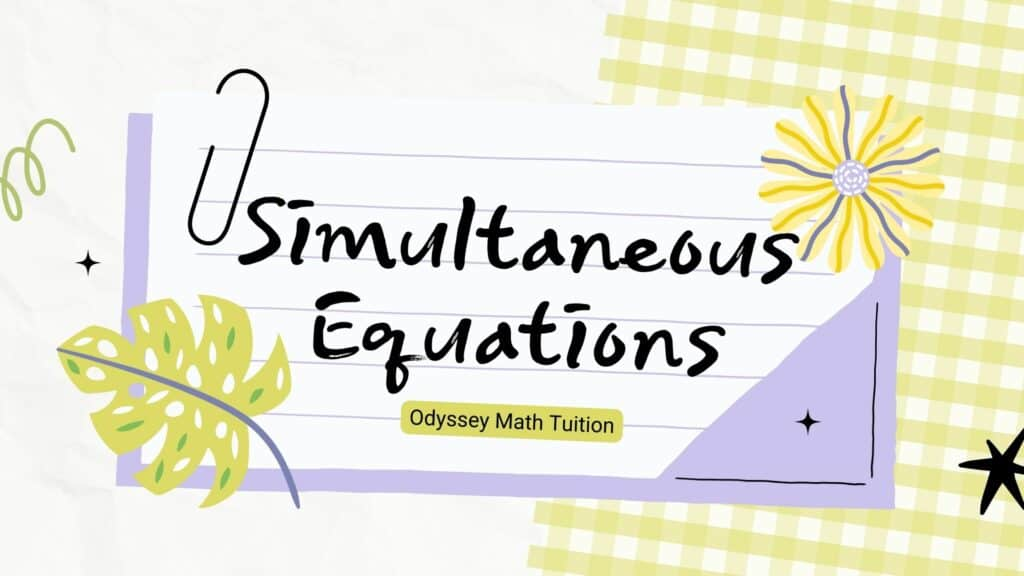# Simultaneous Equations

Simultaneous Equations are two or more algebraic equations that share variables, quite commonly, x and y. In this post, you will understand how to solve simultaneous equations involving non linear equations using the substitution method.